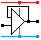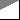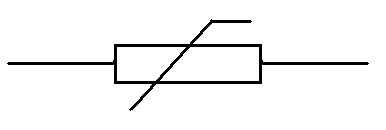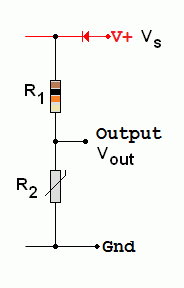Home

rOm
Quest
Glossary

Random
Page
Search
Site
Lush
Sim
Class
Subject
Images

Help
FAQ
Sign
Up
Log
In
 GCSE     Resistors     BS1852     Colour Codes     E24     LDR     Parallel     PIVR Calculations     Potentiometer     Puzzles     Series     >Thermistor<     Timing     Types     Voltage Divider

# Resistors Thermistor

Site for Eduqas/WJEC - Go to the AQA site.

GCSE    ResistorsQuestions 0 to 27   -->  View All

A thermistor is an analogue, passive, resistive, input transducer used to measure temperature.

## Temperature Dependent Resistor (TDR)Negative temperature coefficient resistors (NTC Resistors)

• As the temperature rises, the thermistor resistance drops.
• At low temperatures, few electrons are free to move in the semiconductor material.
• As the temperature rises, increased thermal vibrations cause more electrons to break free and flow.
• Thermistors are used to measure temperature.
• They are fairly slow to react.
• Measurement circuits must be designed with care because the current flowing in the TDR must not significantly heat it up.
• Note that devices like incandescent bulbs behave in the opposite way.
They increase their resistance as the temperature rises.
This is a positive temperature coefficient.

## Thermistor Voltage Divider• Vout = Vs x R2 / (R1 + R2)

## Log Graphs

The thermistor resistance changes over such a large range that normal graphs are not useful. Log graphs solve the problem. Here is an example based on the data sheet for a thermistor with 10K at 25 C.• At 50 the resistance is 25kΩ
• At 250 the resistance is 10kΩ
• When the resistance is 15kΩ the temperature is about 150
• When the resistance is 8kΩ the temperature is 300
• At 1000 the resistance is about 650Ω

## Calculating a Safe Series Resistor

If possible, three design aims need to be met.

1. The current through the thermistor must be small so it does not heat up and alter the temperature you are trying to measure. (Alternatively, the circuit could be turned on for a short time so the thermistor does not have time to heat up.)
2. When the thermistor gets hot, its resistance drops close to zero. Under these conditions, the current must not exceed the maximum safe value for the thermistor.
3. The series resistor value should be similar to the thermistor resistance at the temperature we are trying to detect.

For example in a refrigerator, the cooling pump might come on if the temperature rises above 5 Celsius. From the graph above, the thermistor resistance is about 25kΩ. A 27kΩ series resistor could be used. In a 12 volt circuit, at 50, the current flowing would be ...

I = V / R

I = 12 / ( 25 + 27 )x103

I = 0.23 mA

This current would not have much of a heating effect.

The series resistor is similar in value to the thermistor at 50C.

At much higher temperatures the current would still be less than 12 / 27k   =   0.444mA.

If the thermistor has a maximum heat dissipation of 0.5 Watts and the power supply voltage is 12V, at what temperature does it become impossible to meet all three design requirements? You can assume that the circuit is turned on for a very short time so the heating effect of the current flowing can be ignored.

Subject Name     Level     Topic Name     Question Heading     First Name Last Name Class ID     User ID

 CloseEscapeX Q: qNum of last_q     Q ID: Question ID         Score: num correct/num attempts         Date Done

Question Text

image url

Help Text
Debug

• You can attempt a question as many times as you like.
• If you are logged in, your first attempt, each day, is logged.
• To improve your scores, come back on future days, log in and re-do the questions that caused you problems.
• In the grade book, you can delete your answers for a topic before re-doing the questions. Avoid deleting unless you intend re-doing the questions very soon.